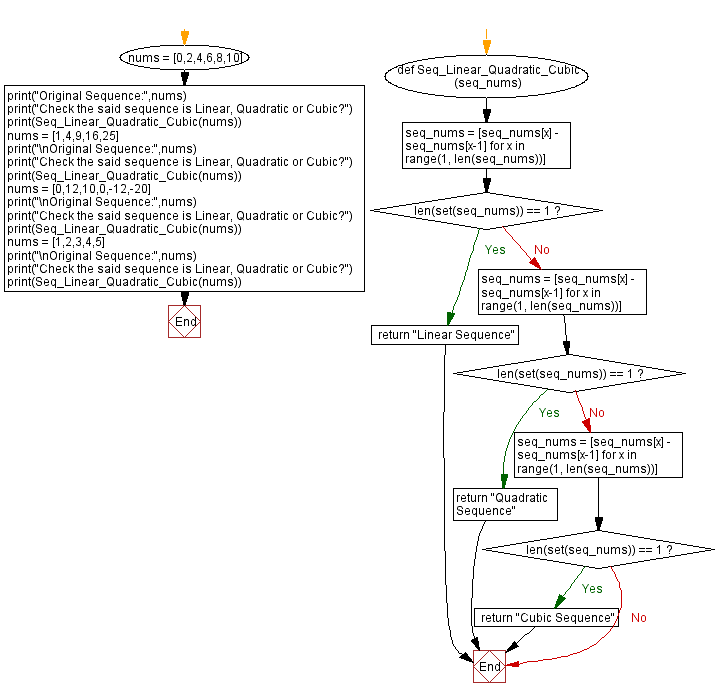﻿ Python: Check whether a given sequence is linear, quadratic or cubic - w3resource# Python: Check whether a given sequence is linear, quadratic or cubic

## Python Basic - 1: Exercise-105 with Solution

Write a Python program to check whether a given sequence is linear, quadratic or cubic.

Sequences are sets of numbers that are connected in some way.
Linear sequence:
A number pattern which increases or decreases by the same amount each time is called a linear sequence. The amount it increases or decreases by is known as the common difference.
In quadratic sequence, the difference between each term increases, or decreases, at a constant rate.
Cubic sequence:
Sequences where the 3rd difference are known as cubic sequence.

Sample Solution:

Python Code:

``````def Seq_Linear_Quadratic_Cubic(seq_nums):
seq_nums = [seq_nums[x] - seq_nums[x-1] for x in range(1, len(seq_nums))]
if len(set(seq_nums)) == 1: return "Linear Sequence"
seq_nums = [seq_nums[x] - seq_nums[x-1] for x in range(1, len(seq_nums))]
if len(set(seq_nums)) == 1: return "Quadratic Sequence"
seq_nums = [seq_nums[x] - seq_nums[x-1] for x in range(1, len(seq_nums))]
if len(set(seq_nums)) == 1: return "Cubic Sequence"

nums = [0,2,4,6,8,10]
print("Original Sequence:",nums)
print("Check the said sequence is Linear, Quadratic or Cubic?")
nums = [1,4,9,16,25]
print("\nOriginal Sequence:",nums)
print("Check the said sequence is Linear, Quadratic or Cubic?")
nums = [0,12,10,0,-12,-20]
print("\nOriginal Sequence:",nums)
print("Check the said sequence is Linear, Quadratic or Cubic?")
nums = [1,2,3,4,5]
print("\nOriginal Sequence:",nums)
print("Check the said sequence is Linear, Quadratic or Cubic?")
``````

Sample Output:

```Original Sequence: [0, 2, 4, 6, 8, 10]
Check the said sequence is Linear, Quadratic or Cubic?
Linear Sequence

Original Sequence: [1, 4, 9, 16, 25]
Check the said sequence is Linear, Quadratic or Cubic?

Original Sequence: [0, 12, 10, 0, -12, -20]
Check the said sequence is Linear, Quadratic or Cubic?
Cubic Sequence

Original Sequence: [1, 2, 3, 4, 5]
Check the said sequence is Linear, Quadratic or Cubic?
Linear Sequence
```

Flowchart:Python Code Editor:

Have another way to solve this solution? Contribute your code (and comments) through Disqus.

What is the difficulty level of this exercise?

Test your Programming skills with w3resource's quiz.

﻿

## Python: Tips of the Day

What is the difference between Python's list methods append and extend?

append: Appends object at the end.

```x = [1, 2, 3]
x.append([4, 5])
print (x)
```

Output:

```[1, 2, 3, [4, 5]]
```

extend: Extends list by appending elements from the iterable.

```x = [1, 2, 3]
x.extend([4, 5])
print (x)
```

Output:

```[1, 2, 3, 4, 5]
```

Ref: https://bit.ly/2AZ6ZFq